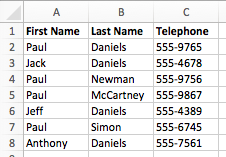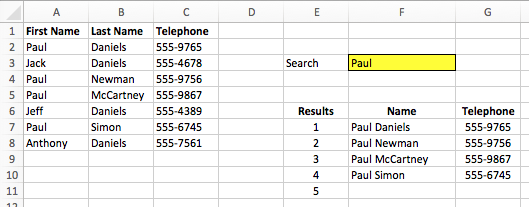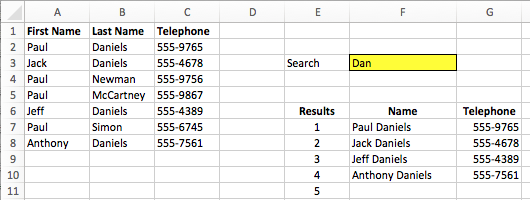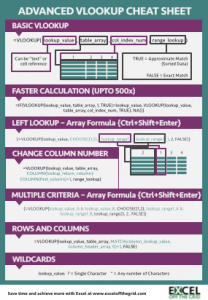Get our FREE VBA eBook of the 30 most useful Excel VBA macros.

Automate Excel so that you can save time and stop doing the jobs a trained monkey could do.

# VLOOKUP: List all the matching itemsIn the previous post in this series, Mastering VLOOKUP, we learned how to look up the 2nd, 3rd, 4th or nth item from a data set.  Today, we will be expanding on that by looking at how to return all the matching values in the dataset.  Plus, we will combine this with partial matches, so that we can create a full powerful search function.

## List all the matching items

VLOOKUP is great when you have unique data, but that doesn’t always happen.  Let’s go back to an example used in a previous post, look at the screenshot below.There 4 different people called Paul who work for your company.  The last time we used this example we knew Paul’s last name.  Imagine that this time, somebody has asked for Paul’s telephone number, but they can’t remember his last name.  Which Paul?  A basic VLOOKUP by itself would be useless as only the first Paul, Paul Daniels’, telephone number would be returned.  We need to be able to list all the matching items.

We can use the formula introduced in the previous post to return a complete list of all Pauls.Cell G7 includes the following formula:

```{=IFERROR(VLOOKUP(SMALL(IF(IF(ISERROR(SEARCH(\$F\$3,\$A\$2:\$A\$8&" "&\$B\$2:\$B\$8)),
FALSE,TRUE),ROW(\$A\$2:\$A\$8)),\$E7),CHOOSE({1,2},ROW(\$A\$2:\$A\$8),
\$C\$2:\$C\$8),2,0),"")}```

This formula has been copied down to G8-G11.

## How does this formula work?

Let’s break down this formula bit by bit.

```{=IFERROR(VLOOKUP(SMALL(IF(IF(ISERROR(SEARCH(\$F\$3,\$A\$2:\$A\$8&" "&\$B\$2:\$B\$8)),
FALSE,TRUE),ROW(\$A\$2:\$A\$8)),\$E7),CHOOSE({1,2},ROW(\$A\$2:\$A\$8),
\$C\$2:\$C\$8),2,0),"")}```

Remember, this is an array formula, so you need to use Ctrl+Shift+Enter to use the formula.

SEARCH Function

```{=IFERROR(VLOOKUP(SMALL(IF(IF(ISERROR(SEARCH(\$F\$3,\$A\$2:\$A\$8&" "&\$B\$2:\$B\$8)),
FALSE,TRUE),ROW(\$A\$2:\$A\$8)),\$E7),CHOOSE({1,2},ROW(\$A\$2:\$A\$8),
\$C\$2:\$C\$8),2,0),"")}```

The SEARCH function is used to find a piece of text within another piece of text.  The key difference between SEARCH and FIND is that SEARCH is not case sensitive.  Cell F3 contains the search value; “Paul”.

Cells A2-A8 is combined with Cells B2-B8 to create a full name.  The array of names {Paul Daniels, Jack Daniels, Paul Newman, Paul McCartney, Jeff Daniels, Paul Simon, Anthony Daniels}.  The result of the SEARCH function is the position of the matched string.  If no match is found the #VALUE! error is returned.  When using “Paul” as an example, he returned result is {1, #VALUE!, 1, 1, #VALUE!, 1, #VALUE!}

ISERROR Function

```{=IFERROR(VLOOKUP(SMALL(IF(IF(ISERROR(SEARCH(\$F\$3,\$A\$2:\$A\$8&" "&\$B\$2:\$B\$8)),
FALSE,TRUE),ROW(\$A\$2:\$A\$8)),\$E7),CHOOSE({1,2},ROW(\$A\$2:\$A\$8),
\$C\$2:\$C\$8),2,0),"")}```

The ISERROR function surrounds the returned result of the SEARCH function.  This function will turn any error into a TRUE and any non-ERROR into a FALSE.  The returned result is now converted to {FALSE, TRUE, FALSE, FALSE, TRUE, FALSE, TRUE}

IF Function

```{=IFERROR(VLOOKUP(SMALL(IF(IF(ISERROR(SEARCH(\$F\$3,\$A\$2:\$A\$8&" "&\$B\$2:\$B\$8)),
FALSE,TRUE),ROW(\$A\$2:\$A\$8)),\$E7),CHOOSE({1,2},ROW(\$A\$2:\$A\$8),
\$C\$2:\$C\$8),2,0),"")}```

The double IF function is used to invert the TRUE/FALSE values, then if TRUE retrieve the ROW number.

The IF surrounding ISERROR function will return {TRUE, FALSE, TRUE, TRUE, FALSE, TRUE, FALSE}.  Then IF surrounding that IF will return {2, FALSE, 4, 5, FALSE, 7, FALSE}

SMALL Function

```{=IFERROR(VLOOKUP(SMALL(IF(IF(ISERROR(SEARCH(\$F\$3,\$A\$2:\$A\$8&" "&\$B\$2:\$B\$8)),
FALSE,TRUE),ROW(\$A\$2:\$A\$8)),\$E7),CHOOSE({1,2},ROW(\$A\$2:\$A\$8),
\$C\$2:\$C\$8),2,0),"")}```

The SMALL function is used to return the nth lookup, based on value in Cells E7-E11.

The rest of the formula

```{=IFERROR(VLOOKUP(SMALL(IF(IF(ISERROR(SEARCH(\$F\$3,\$A\$2:\$A\$8&" "&\$B\$2:\$B\$8)),
FALSE,TRUE),ROW(\$A\$2:\$A\$8)),\$E7),CHOOSE({1,2},ROW(\$A\$2:\$A\$8),
\$C\$2:\$C\$8),2,0),"")}```

The red section above was explained in the previous post, so please refer there to get a fuller description.

IFERROR Function

The IFERROR Function is used to return a blank cell for any values which are not matched.

```{=IFERROR(VLOOKUP(SMALL(IF(IF(ISERROR(SEARCH(\$F\$3,\$A\$2:\$A\$8&" "&\$B\$2:\$B\$8)),
FALSE,TRUE),ROW(\$A\$2:\$A\$8)),\$E7),CHOOSE({1,2},ROW(\$A\$2:\$A\$8),
\$C\$2:\$C\$8),2,0),"")}```

The result

When copied down from Cell G7-G11 the VLOOKUP will return a list of all the matching items.

## Returning the full name

We may have found the telephone numbers of every Paul, but we also need to retrieve the full name.  The formula in F7 is similar to G7 (with the differences highlighted below).  Our formula will return the first name and last name separated by a space.

```=IFERROR(VLOOKUP(SMALL(IF(IF(ISERROR(SEARCH(\$F\$3,\$A\$2:\$A\$8&" "&\$B\$2:\$B\$8)),
FALSE,TRUE),ROW(\$A\$2:\$A\$8)),\$E7),CHOOSE({1,2},ROW(\$A\$2:\$A\$8),
\$A\$2:\$A\$8&" "&\$B\$2:\$B\$8),2,0),"")```

## Returning partial matches

As we have used the SEARCH function it is capable of returning partial matches.  If we search for “Dan” it will provide a list of all the matching results.This formula can also work with wildcards.  If we search for “paul*m” there are 3 matching resultsDownload the Advanced VLOOKUP Cheat Sheet.  It includes most of the tips and tricks we’ve covered in this series, including faster calculations, multiple criteria, left lookup and much more.## Other posts in the Mastering VLOOKUP Series

Save

Hey, I’m Mark, and I run Excel Off The Grid.

My parents tell me that at the age of 7 I declared I was going to become a qualified accountant. I was either psychic or had no imagination, as that is exactly what happened. However, it wasn't until I was 35 that my journey really began.

In 2015, I started a new job, for which I was regularly working after 10pm. As a result, I rarely saw my children during the week. So, I started searching for the secrets to automating Excel. I discovered that by building a small number of simple tools, I could combine them together in different ways to automate nearly all my regular tasks. This meant I could work less hours (and I got pay raises!). Today, I teach these techniques to other professionals in our training program so they too can spend less time at work (and more time with their children and doing the things they love).

I'm guessing the examples in this post don't exactly match your situation. We all use Excel differently, so it's impossible to write a post that will meet everybody's needs. By taking the time to understand the techniques and principles in this post (and elsewhere on this site), you should be able to adapt it to your needs.

But, if you're still struggling you should:

1. Read other blogs, or watch YouTube videos on the same topic. You will benefit much more by discovering your own solutions.
2. Ask the 'Excel Ninja' in your office. It's amazing what things other people know.
3. Ask a question in a forum like Mr Excel, or the Microsoft Answers Community. Remember, the people on these forums are generally giving their time for free. So take care to craft your question, make sure it's clear and concise.  List all the things you've tried, and provide screenshots, code segments and example workbooks.
4. Use Excel Rescue, who are my consultancy partner. They help by providing solutions to smaller Excel problems.

What next?
Don't go yet, there is plenty more to learn on Excel Off The Grid.  Check out the latest posts:

## 2 thoughts on “VLOOKUP: List all the matching items”

1.Dariusz says:

I like it

•Excel Off The Grid says:

Thank you 🙂